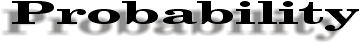Probability is another way of looking at the likelihood of an event. It compares the number of ways an event can happen to the number of total possible outcomes.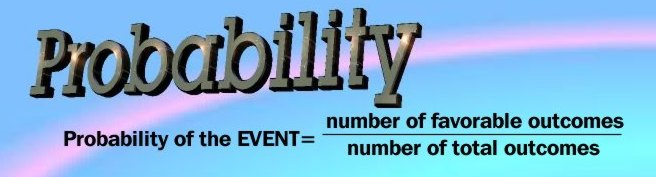Pick a number from 1 to 10. What is the probability that you picked an odd number? There are five odd numbers between 1 and 10. The probability is 5:10. Or you can express it as a fraction: 5/10. Since it's a fraction, why not reduce it? The probability that you will pick an odd number is 1/2. Probability can also be expressed as a percent...1/2=50% Or as a decimal...1/2=50%=.5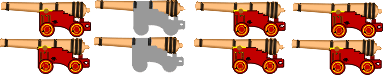There are 8 cannons on the wall of a fortress. Two of the cannons are empty and 6 are ready to fire. What is the probability that a cannon selected at random will fire?In this case, there are 6 favorable outcomes (cannons that will fire) and a total of 8 outcomes all together. The probability is 6 to 8. Written as a fraction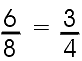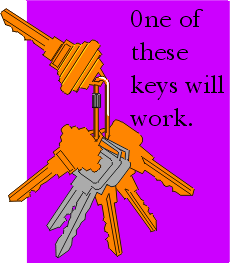What is the probability that a key chosen at random will be the one that opens the lock?

Permutations

 writing: Kirsten Anderson art: mC